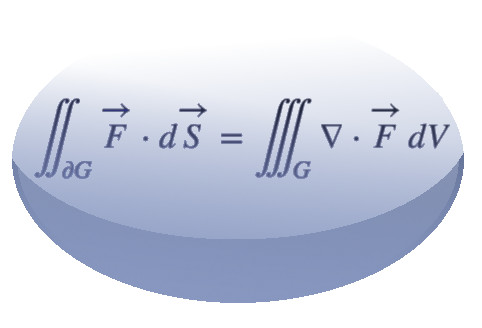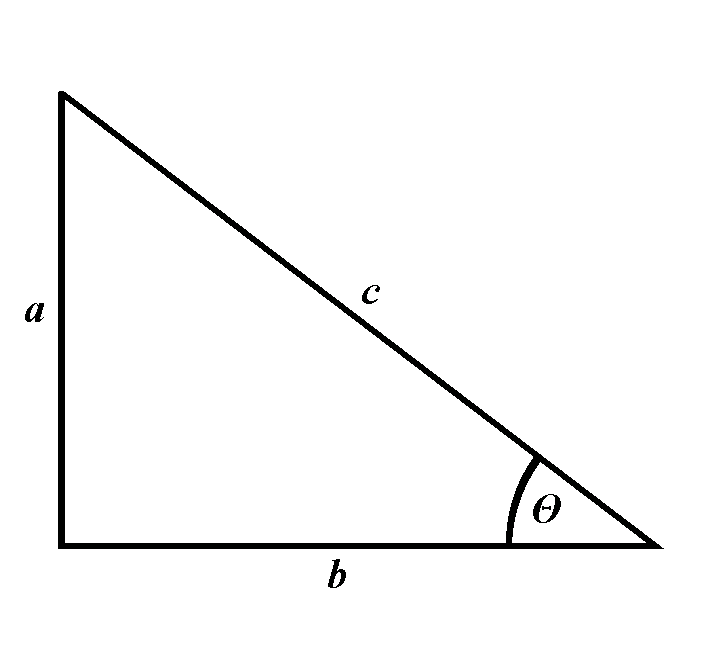# Calculus## Introduction

All of the texts are followed by online quizzes. Once you solve the quiz, you will see your score and official solutions. You may work on the quiz multiple times. Our database of problems is constantly growing, and you can expect a new quiz after you solve the old one. Make sure you are logged in to take the full advantage of our adaptive learning system.

## Contents

### Single variable calculus

$\quad\quad\quad$

## Formulas

### Precalculus and trigonometry

 $$\displaystyle (a+b)^2=a^2+2ab+b^2$$ $$\displaystyle (a+b)(c+d)=ac+ad+bc+bd$$ $$\displaystyle n!=1\cdot2\cdots\cdot (n-1)\cdot n$$ $$\displaystyle \binom{n}k=\frac{n!}{k!(n-k)!}$$ $$\displaystyle (a+b)^n=\sum_{i=0}^n\binom{n}ia^ib^{n-i}$$ $$\displaystyle a^2-b^2=(a+b)(a-b)$$ $$\displaystyle (ab)^n=a^nb^n$$ $$\displaystyle \left(a^x\right)^y=a^{xy}$$ $$\displaystyle e^{\ln x}=x$$ $$\displaystyle a^x=e^{x\ln a}$$ $$\displaystyle \ln(ab)=\ln a+\ln b$$ $$\displaystyle \ln\left(a^k\right)=k\ln a$$ $\quad$$$\displaystyle \sin\theta=\frac{a}{c}$$ $$\displaystyle \cos\theta=\frac{b}{c}$$ $\quad$ $$\displaystyle \sin^2\alpha+\cos^2\alpha=1$$ $$\displaystyle \tan\alpha=\frac{\sin\alpha}{\cos\alpha}$$ $$\displaystyle \cot\alpha=\frac{\cos\alpha}{\sin\alpha}$$ $$\displaystyle \sec \alpha=\frac1{\cos \alpha}$$ $$\displaystyle \sec^2\alpha=1+\tan^2\alpha$$ $$\displaystyle \cos(\alpha+\beta)=\cos\alpha\cos\beta-\sin\alpha\sin\beta$$ $$\displaystyle \sin(\alpha+\beta)=\sin\alpha\cos\beta+\cos\alpha\sin\beta$$ $$\displaystyle \cos(2\alpha)=\cos^2\alpha-\sin^2\alpha$$ $$\displaystyle \sin(2\alpha)=2\sin\alpha\cos\alpha$$ $$\displaystyle \cos^2\alpha=\frac{1+\cos(2\alpha)}2$$ $$\displaystyle \sin^2\alpha=\frac{1-\cos(2\alpha)}2$$

### Derivatives

 $$\displaystyle \frac{d}{dx} C=0$$ $$\displaystyle \frac{d}{dx} x=1$$ $$\displaystyle \frac{d}{dx} x^k=kx^{k-1}$$, for $$k\in\mathbb R$$ $$\displaystyle \frac{d}{dx} \ln x=\frac1x$$ $$\displaystyle \frac{d}{dx} e^x=e^x$$ $$\displaystyle \frac{d}{dx} a^x=a^x\ln a$$, for $$a> 0$$ $\quad$ $$\displaystyle \frac{d}{dx}\sin x=\cos x$$ $$\displaystyle\frac{d}{dx}\cos x=-\sin x$$ $$\displaystyle \frac{d}{dx} \tan x=\frac1{\cos^2x}$$ $$\displaystyle \frac{d}{dx} \cot x=-\frac1{\sin^2x}$$ $$\displaystyle \frac{d}{dx} \arcsin x=\frac1{\sqrt{1-x^2}}$$ $$\displaystyle \frac{d}{dx}\arccos x=-\frac1{\sqrt{1-x^2}}$$ $\quad$ $$\displaystyle \frac{d}{dx}\arctan x=\frac1{1+x^2}$$ $$\displaystyle \frac{d}{dx}\sinh x=\cosh x$$ $$\displaystyle \frac{d}{dx}\cosh x=\sinh x$$ $$\displaystyle \frac{d}{dx}\tanh x=\frac1{\cosh^2 x}$$ $$\displaystyle \frac{d}{dx}\coth x=-\frac1{\sinh^2 x}$$ $$\displaystyle \left(f(x)\cdot g(x)\right)^{\prime}=f^{\prime}(x)g(x)+f(x)g^{\prime}(x)$$ $$\displaystyle \left(\frac{f(x)}{g(x)}\right)^{\prime}=\frac{f^{\prime}(x)g(x)-f(x)g^{\prime}(x)}{\left(g(x)\right)^2}$$ $$\displaystyle \left( f(g(x))\right)^{\prime}=f^{\prime}(g(x))\cdot g^{\prime}(x)$$

### Integrals

 $$\displaystyle \int x^p\,dx= \frac{x^{p+1}}{p+1}+C$$, for $$p\neq -1$$ $$\displaystyle \int \frac1x\,dx= \ln\left|x\right|+C$$ $$\displaystyle\int e^x\,dx= e^x+C$$ $$\displaystyle\int a^x\,dx= \frac{a^x}{\ln a}+C$$, for $$a> 0$$ and $$a\neq 1$$ $$\displaystyle\int \ln x\,dx=x\ln x-x+C$$ $$\displaystyle\int \cos x\,dx=\sin x+C$$ $$\displaystyle\int \sin x\,dx=-\cos x+C$$ $$\displaystyle \int \frac1{\cos^2x}\,dx=\tan x+C$$ $$\displaystyle \int \frac1{\sin^2x}\,dx=-\cot x+C$$ $$\displaystyle \int \sec x\cdot \tan x\,dx=\sec x+C$$ $$\displaystyle \int\tan x\,dx=-\ln\left|\cos x\right|+C$$ $$\displaystyle \int\cot x\,dx=\ln\left|\sin x\right|+C$$ $$\displaystyle \int \arcsin x\,dx=x\arcsin x+\sqrt{1-x^2}+C$$ $$\displaystyle \int \arctan x\,dx=x\arctan x-\frac12\ln\left(1+x^2\right)+C$$ $\quad$ $$\displaystyle \int e^{ax}\sin(bx)\,dx =\frac{e^{ax}\left(a\sin(bx)-b\cos(bx)\right)}{a^2+b^2}+C$$ $$\displaystyle \int e^{ax}\cos(bx)\,dx=\frac{e^{ax}\left(a\cos(bx)+b\sin(bx)\right)}{a^2+b^2}+C$$ $$\displaystyle \int \frac{dx}{x^2+a^2}=\frac1a\arctan\frac{x}{a}+C$$ $$\displaystyle \int \frac{dx}{x^2-a^2}=\frac1{2a}\ln\left|\frac{x-a}{x+a}\right|+C$$ $$\displaystyle \int \frac{dx}{\sqrt{a^2-x^2}}=\arcsin \frac{x}{a}+C$$ $$\displaystyle \int\frac{dx}{\sqrt{x^2\pm a^2}}=\ln\left|x+\sqrt{x^2\pm a^2}\right|+C$$ $$\displaystyle \int\frac{dx}{\sqrt{x^2\pm a^2}^3}=\pm\frac{x}{a^2\sqrt{x^2\pm a^2}}+C$$ $$\displaystyle \int \sqrt{x^2\pm a^2}\,dx=\pm\frac{a^2}2\ln\left|x +\sqrt{x^2\pm a^2}\right|$$ $$\displaystyle+\frac{x}2\sqrt{x^2\pm a^2}+C$$ $$\displaystyle \int \sqrt{x^2\pm a^2}^3\,dx=\frac{x}8\left(2x^2\pm5a^2\right)\sqrt{x^2\pm a^2}$$ $$\displaystyle+\frac{3a^4}8\ln\left|x+\sqrt{x^2\pm a^2}\right|+ C$$ $$\displaystyle \int_{-\infty}^{+\infty}e^{-x^2}\,dx=\sqrt{\pi}$$ $$\displaystyle \int_0^{+\infty}\frac{\sin x}x\,dx=\frac{\pi}2$$ $$\displaystyle \int_0^{\frac{\pi}2}\sin^{2n}x\,dx=\frac{(2n-1)!!}{(2n)!!}\cdot\frac{\pi}2$$, for $$n\in\mathbb N\cup\{0\}$$ $$\displaystyle \int_0^{\frac{\pi}2}\sin^{2n+1}x\,dx=\frac{(2n)!!}{(2n+1)!!}$$, for $$n\in\mathbb N\cup \{0\}$$

2005-2021 IMOmath.com | imomath"at"gmail.com | Math rendered by MathJax